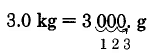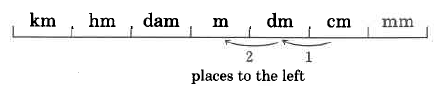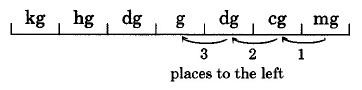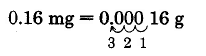$$\newcommand{\id}{\mathrm{id}}$$ $$\newcommand{\Span}{\mathrm{span}}$$ $$\newcommand{\kernel}{\mathrm{null}\,}$$ $$\newcommand{\range}{\mathrm{range}\,}$$ $$\newcommand{\RealPart}{\mathrm{Re}}$$ $$\newcommand{\ImaginaryPart}{\mathrm{Im}}$$ $$\newcommand{\Argument}{\mathrm{Arg}}$$ $$\newcommand{\norm}{\| #1 \|}$$ $$\newcommand{\inner}{\langle #1, #2 \rangle}$$ $$\newcommand{\Span}{\mathrm{span}}$$

# 9.2: The Metric System of Measurement

$$\newcommand{\vecs}{\overset { \rightharpoonup} {\mathbf{#1}} }$$ $$\newcommand{\vecd}{\overset{-\!-\!\rightharpoonup}{\vphantom{a}\smash {#1}}}$$$$\newcommand{\id}{\mathrm{id}}$$ $$\newcommand{\Span}{\mathrm{span}}$$ $$\newcommand{\kernel}{\mathrm{null}\,}$$ $$\newcommand{\range}{\mathrm{range}\,}$$ $$\newcommand{\RealPart}{\mathrm{Re}}$$ $$\newcommand{\ImaginaryPart}{\mathrm{Im}}$$ $$\newcommand{\Argument}{\mathrm{Arg}}$$ $$\newcommand{\norm}{\| #1 \|}$$ $$\newcommand{\inner}{\langle #1, #2 \rangle}$$ $$\newcommand{\Span}{\mathrm{span}}$$ $$\newcommand{\id}{\mathrm{id}}$$ $$\newcommand{\Span}{\mathrm{span}}$$ $$\newcommand{\kernel}{\mathrm{null}\,}$$ $$\newcommand{\range}{\mathrm{range}\,}$$ $$\newcommand{\RealPart}{\mathrm{Re}}$$ $$\newcommand{\ImaginaryPart}{\mathrm{Im}}$$ $$\newcommand{\Argument}{\mathrm{Arg}}$$ $$\newcommand{\norm}{\| #1 \|}$$ $$\newcommand{\inner}{\langle #1, #2 \rangle}$$ $$\newcommand{\Span}{\mathrm{span}}$$

Learning Objectives

• be more familiar with some of the advantages of the base ten number system
• know the prefixes of the metric measures
• be familiar with the metric system of measurement
• be able to convert from one unit of measure in the metric system to another unit of measure

## The Advantages of the Base Ten Number System

The metric system of measurement takes advantage of our base ten number sys­tem. The advantage of the metric system over the United States system is that in the metric system it is possible to convert from one unit of measure to another simply by multiplying or dividing the given number by a power of 10. This means we can make a conversion simply by moving the decimal point to the right or the left.

## Prefixes

Common units of measure in the metric system are the meter (for length), the liter (for volume), and the gram (for mass). To each of the units can be attached a prefix. The metric prefixes along with their meaning are listed below.

Metric Prefixes

kilo: thousand
deci: tenth
hecto: hundred
centi: hundredth
deka: ten
milli: thousandth

For example, if length is being measured,

1 kilometer is equivalent to 1000 meters.
1 centimeter is equivalent to one hundredth of a meter.
1 millimeter is equivalent to one thousandth of a meter.

## Conversion from One Unit to Another Unit

Let's note three characteristics of the metric system that occur in the metric table of measurements.

1. In each category, the prefixes are the same.
2. We can move from a larger to a smaller unit of measure by moving the decimal point to the right.
3. We can move from a smaller to a larger unit of measure by moving the decimal point to the left.

The following table provides a summary of the relationship between the basic unit of measure (meter, gram, liter) and each prefix, and how many places the decimal point is moved and in what direction.

kilo hecto deka unit deci centi milli

 Basic Unit to Prefix Move the Decimal Point unit to deka 1 to 10 1 place to the left unit to hector 1 to 100 2 places to the left unit to kilo 1 to 1,000 3 places to the left unit to deci 1 to 0.1 1 place to the right unit to centi 1 to 0.01 2 places to the right unit to milli 1 to 0.001 3 places to the right

## Conversion Table

Listed below, in the unit conversion table, are some of the common metric units of measure.

 Unit Conversion Table Length $$\text{1 kilometer (km) = 1,000 meters } (m)$$ $$1,000 \times 1\text{m}$$ $$\text{1 hectometer (hm) = 100 meters}$$ $$100 \times 1 \text{m}$$ $$\text{1 dekameter (dam) = 10 meters}$$ $$10 \times 1 \text{m}$$ $$\text{1 meter (m)}$$ $$1 \times 1 \text{m}$$ $$\text{1 decimeter (dm) = } \dfrac{1}{10} \text{ meter}$$ $$.1 \times 1 \text{m}$$ $$\text{1 centimeter (cm) = } \dfrac{1}{100} \text{ meter}$$ $$.01 \times 1 \text{m}$$ $$\text{1 millimeter (mm) = } \dfrac{1}{1,000} \text{ meter}$$ $$.001 \times 1 \text{m}$$ Mass $$\text{1 kilogram (kg) = 1,000 grams } (g)$$ $$1,000 \times 1\text{g}$$ $$\text{1 hectogram (hg) = 100 grams}$$ $$100 \times 1 \text{g}$$ $$\text{1 dekagram (dag) = 10 grams}$$ $$10 \times 1 \text{g}$$ $$\text{1 gram (g)}$$ $$1 \times 1 \text{g}$$ $$\text{1 decigram (dg) = } \dfrac{1}{10} \text{ gram}$$ $$.1 \times 1 \text{g}$$ $$\text{1 centigram (cg) = } \dfrac{1}{100} \text{ gram}$$ $$.01 \times 1 \text{g}$$ $$\text{1 milligram (mg) = } \dfrac{1}{1,000} \text{ gram}$$ $$.001 \times 1 \text{g}$$ Volume $$\text{1 kiloliter (kL) = 1,000 liters } (L)$$ $$1,000 \times 1\text{L}$$ $$\text{1 hectoliter (hL) = 100 liters}$$ $$100 \times 1 \text{L}$$ $$\text{1 dekaliter (daL) = 10 liters}$$ $$10 \times 1 \text{L}$$ $$\text{1 liter (L)}$$ $$1 \times 1 \text{L}$$ $$\text{1 deciliter (dL) = } \dfrac{1}{10} \text{ liter}$$ $$.1 \times 1 \text{L}$$ $$\text{1 centiliter (cL) = } \dfrac{1}{100} \text{ liter}$$ $$.01 \times 1 \text{L}$$ $$\text{1 milliliter (mL) = } \dfrac{1}{1,000} \text{ liter}$$ $$.001 \times 1 \text{L}$$ Time Same as the United States system

Distinction Between Mass and Weight
There is a distinction between mass and weight. The weight of a body is related to gravity whereas the mass of a body is not. For example, your weight on the earth is different than it is on the moon, but your mass is the same in both places. Mass is a measure of a body's resistance to motion. The more massive a body, the more resistant it is to motion. Also, more massive bodies weigh more than less massive bodies.

Converting Metric Units
To convert from one metric unit to another metric unit:

1. Determine the location of the original number on the metric scale (pictured in each of the following examples).
2. Move the decimal point of the original number in the same direction and same number of places as is necessary to move to the metric unit you wish to go to.

We can also convert from one metric unit to another using unit fractions. Both methods are shown in Sample Set A.

Sample Set A

Convert 3 kilograms to grams.

Solution

a. 3 kg can be written as 3.0 kg. Then,Thus, $$\text{3 kg = 3,000 g}$$.

b. We can also use unit fractions to make this conversion.

Since we are converting to grams, and $$\text{1,000 g = 1 kg}$$. we choose the unit fraction $$\dfrac{\text{1,000 g}}{\text{1 kg}}$$ since grams is in the numerator.

$$\begin{array} {rcl} {\text{3 kg}} & = & {\text{3 kg} \cdot \dfrac{\text{1,000 g}}{\text{1 kg}}} \\ {} & = & {3 \cancel{\text{kg}} \cdot \dfrac{\text{1,000 g}}{1 \cancel{\text{kg}}}} \\ {} & = & {3 \cdot 1,000 \text{ g}} \\ {} & = & {3,000 \text{ g}} \end{array}$$

Sample Set A

Convert 67.2 hectoliters to milliliters.

SolutionThus, $$\text{67.2 hL = 6,720,000 mL}$$.

Sample Set A

Convert 100.07 centimeters to meters.

SolutionThus, $$\text{100.07 cm = 1.0007m}$$.

Sample Set A

Convert 0.16 milligrams to grams.

SolutionThus, $$\text{0.16 mg = 0.00016}$$.

Practice Set A

Convert 411 kilograms to grams.

411,000 g

Practice Set A

Convert 5.626 liters to centiliters.

562.6 cL

Practice Set A

Convert 80 milliliters to kiloliters.

0.00008 kL

Practice Set A

Convert 150 milligrams to centigrams.

15 cg

Practice Set A

Convert 2.5 centimeters to meters.

0.025 m

## Exercises

Make each conversion.

Exercise $$\PageIndex{1}$$

87 m to cm

8,700 cm

Exercise $$\PageIndex{2}$$

905 L to mL

Exercise $$\PageIndex{3}$$

16,005 mg to g

16.005 g

Exercise $$\PageIndex{4}$$

48.66 L to dL

Exercise $$\PageIndex{5}$$

11.161 kL to L

11,161 L

Exercise $$\PageIndex{6}$$

521.85 cm to mm

Exercise $$\PageIndex{7}$$

1.26 dag to dg

126 dg

Exercise $$\PageIndex{8}$$

99.04 dam to cm

Exercise $$\PageIndex{9}$$

0.51 kL to daL

5.1 daL

Exercise $$\PageIndex{10}$$

0.17 kL to daL

Exercise $$\PageIndex{11}$$

0.05 m to dm

0.5 dm

Exercise $$\PageIndex{12}$$

0.001 km to mm

Exercise $$\PageIndex{13}$$

8.106 hg to cg

81,060 cg

Exercise $$\PageIndex{14}$$

17.0186 kL to mL

Exercise $$\PageIndex{15}$$

3 cm to m

0.03 m

Exercise $$\PageIndex{16}$$

9 mm to m

Exercise $$\PageIndex{17}$$

4 g to mg

4,000 mg

Exercise $$\PageIndex{18}$$

2 L to kL

Exercise $$\PageIndex{19}$$

6 kg to mg

6,000,000 mg

Exercise $$\PageIndex{20}$$

7 daL to mL

#### Exercises for Review

Exercise $$\PageIndex{21}$$

Find the value of $$\dfrac{5}{8} - \dfrac{1}{3} + \dfrac{3}{4}$$

$$\dfrac{25}{24} = 1 \dfrac{1}{24}$$

Exercise $$\PageIndex{22}$$

Solve the proportion: $$\dfrac{9}{x} = \dfrac{27}{60}$$.

Exercise $$\PageIndex{23}$$

Use the method of rounding to estimate the sum: $$8,226 + 4,118$$.

12,300 (12,344)

Exercise $$\PageIndex{24}$$

Use the clustering method to estimate the sum: $$87 + 121 + 118 + 91 + 92$$.

Exercise $$\PageIndex{25}$$

Convert 3 in. to yd.

$$0.08\overline{3}$$ yard.